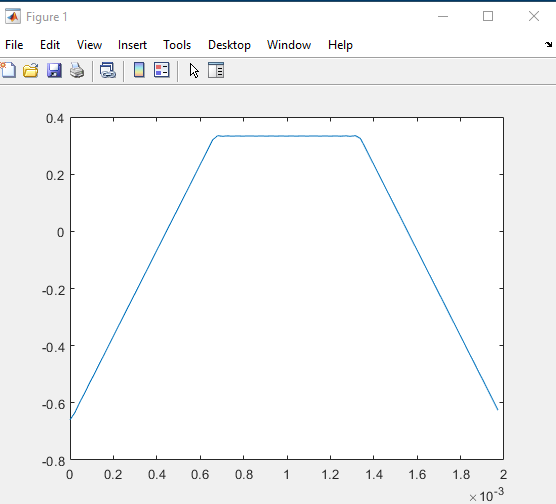Impact-Site-Verification: dbe48ff9-4514-40fe-8cc0-70131430799e

## Search This Blog%% user input

f = 500;                       %fundamental frequency

P = 2000;                      %number of periods

N=40;                          %partial harmonics

%% build 1 period trapezoid wave and plot

Fs = 44100;                    %sampling frequency

x = 0:1/Fs:1/f-1/Fs;           %time vector

T=0;                           %initialize expansion

for n=1:N                      %build 1 period trapezoid wave

A = (3*cos(2*pi*n/3)-3)/(pi^2*n^2);

T = T+A*cos(2*pi*n*f*x);

end

plot(x,T)

%% repeat for P periods and play sound

Tloop = repmat(T,1,P);         %repeat loop

sound(Tloop,Fs)                %play sound